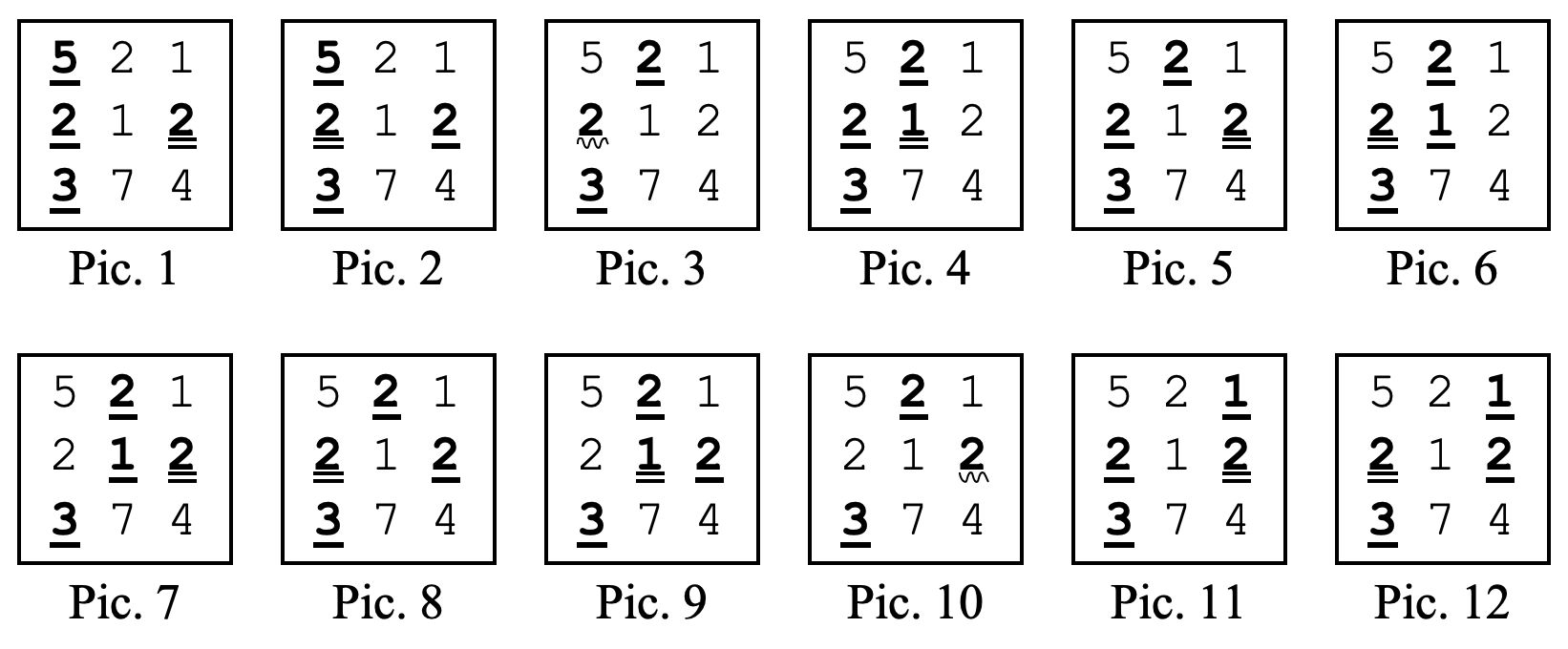시간 제한메모리 제한제출정답맞힌 사람정답 비율
1 초 128 MB0000.000%

## 문제

Dumitru had a very strange dream: he dreamed that he was in a room having its door locked. There he found n boxes each containing exactly m small plates, on each plate being written an integer number greater or equal to 1. He also found a small note containing 2 integer numbers k and l, and specifying the following task:

• Step 1: Pick one plate from the first box, write its number in your notebook, change the number on the plate to 1, and put the plate back into the box. Subsequently, in the same way, pick a single plate from the second box, then one from the third box, and so on till the n-th (last) box, inclusive, each time picking a plate from the respective box, writing its number in your notebook, changing the number on that plate to 1 and then placing the plate back into that box.
• Step 2: After this, in the same manner, pick a single plate from the box n – 1, then one from box n – 2, then one from box n – 3, and so on till the second box, inclusive, each time picking a plate from the respective box.

To unlock the door of the room and to get out, Dumitru needed to find the number T of different ways of picking plates according to the above scenario, such that the product of the numbers that were written in your notebook is divisible by k. Because T could be extremely large, he needed to return the remainder of T when divided by l.

Write a program which will help Dumitru to solve the task.

## 입력

Input contains 2 integer numbers on the first line: n and m, separated by a single space. Second line contains 2 integer numbers k and l separated by a single space. Then n lines follow, each containing m integer numbers separated by single spaces. The first of these lines contains the m numbers written on the plates found in the first box, the second of these lines contains the numbers written on the plates found in the second box, and so on.

## 출력

Output will contain one integer number that is the remainder of T when divided by l.

## 제한

• 3 ≤ n ≤ 200
• 3 ≤ m ≤ 10 000
• 2 ≤ k ≤ 200 000
• 2 ≤ l ≤ 30 000
• Numbers on the plates in boxes have integer values between 1 and 1 000 000 inclusive.

## 예제 입력 1

3 3
12 100
5 2 1
2 1 2
3 7 4


## 예제 출력 1

12


## 힌트

According to the steps described in the task, there are 12 different ways of picking the plates such that the product of numbers written in your notebook is divisible by 12. All 12 ways are shown below, where the numbers of the plates picked in Step 1 are underlined with a single straight line, the numbers of plates picked in Step 2 are underlined with two straight lines, and the numbers of plates picked in both steps are underlined with a curved line.As can be seen, Pic. 1 and Pic. 2 show 2 different ways of picking plates, even if in both cases the same set of plates are picked. In Pic.3 the first plate from the second row is picked in both Step 1 and Step 2, and the product of numbers written in the notebook in this case is thus equal to  , because number 2 on the plate picked in both steps is rewritten with 1 after the plate is picked in Step 1, and thus when it’s selected again in Step 2 the number written on it is 1.